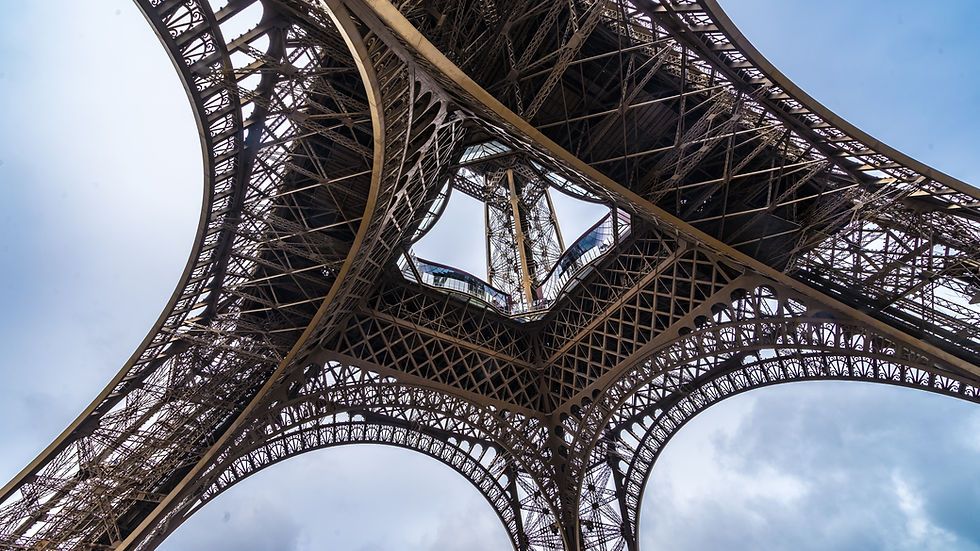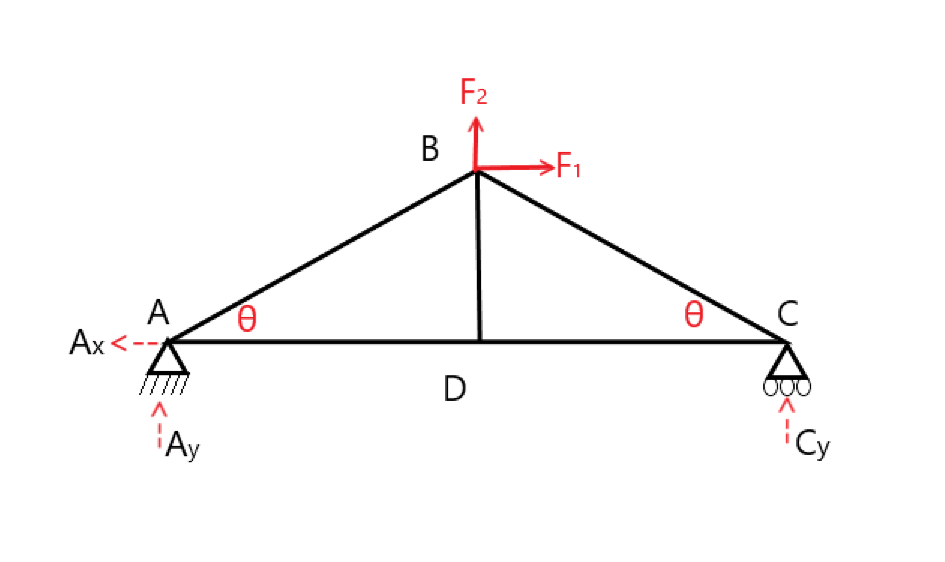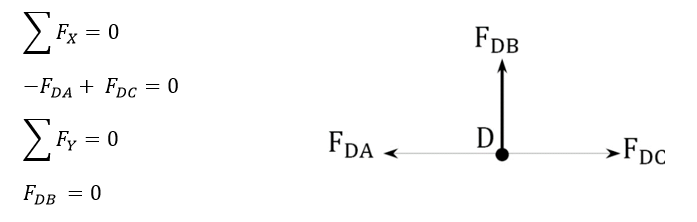top of page
Search
• Abhishek

# Building a structure | Truss

Updated: Apr 23, 2021

How does this structure stand?Eiffel Tower. It's one of the seven wonders of the world.

A beautiful and marvelous structure. But have you ever looked at it and thought, it's nothing but a number of bars connected to each other. Well, technically you are correct. And we have a term for that -Truss.

### Definition:

A truss is a rigid structure that is composed of beams joint to one another. The beams or rods are joined to form a structure that could withstand heavy load and distribute weight.

### Stability of structure:

Any Truss structure formed needs to have stability. The rigidity of the structure is directly dependent on the stability of the structure.

Can we just connect the rods randomly to form a usable structure? How can we make sure that the structure we are building is a stable and rigid structure?

To determine this, we have an equilibrium equation or commonly known as Maxwell Equation.Where, j = number of joints, m = number of members, and r = number of unknown support reactions

### Forces involved:The forces involved in a truss structure are similar to a 2-dimensional structure. The elements are experiencing 2 types of forces, compression, and tension.

Compression: The force which is causing the structural element in this case the beam to undergo compression is known as compressive force.

Tension: The force which causes the structural element to go in tension or causes elongation is known as tension force.

A component in the truss experiences both tension and compression force depending on the point of reference.

A truss can be built in various possible shapes but the force analysis for the complex structures is done by breaking it down to the simpler structure.

### Procedure for Analysis of forces involved in Truss

1. We need to check the stability of the structure before proceeding to the analysis. If the truss is stable and determinate, then we move to the next step.

2. In a truss, there are some components that don’t have any force or reaction carried through them. Such components are called zero-force members. We can ignore these components while calculations.

3. Select a joint or section from the truss such that there are less than two unknown forces to be analyzed.

4. Draw the isolated free-body diagram of the selected joint, and indicate the axial forces in all members meeting at the joint as tensile (i.e. as pulling away from the joint). If this initial assumption is wrong, the determined member axial force will be negative in the analysis, meaning that the member is in compression and not in tension.

5. Apply the two equations Σ Fx = 0 and Σ Fy = 0 to determine the member axial forces.

6. Continue the analysis by proceeding to the next joint with two or fewer unknown member forces.

Let us take a look at the breakdown of forces in a simple truss component.From the above illustration, we can see that the truss has 5 link members, 4 joints, and 2 support members.

First, we will determine the forces at support reaction by applying the equation for static equilibrium.With help of this equation, we will determine the support reactions, later we'll do an analysis on joint A and determine the forces involved.Similarly, applying the equation of static equilibrium on joint D and BSolving the above equations we will get the forces in each component of the truss.

Summary:• A truss is a rigid structure built with several rods/bars.

• The angle of the joint between the bars affects the stability and load-carrying ability of the structure.

• The nature of any truss structure can be determined using Maxwell's equation.

• Support reactions of the structure affect the force distribution.

• If a truss system is rigid and determinant, the sum of all the forces at a node or joint will be zero.

You must have seen a truss structure many times in your life. But, did you know it was called truss or how important these structures are? Well, now you know.

To know more about such amazing structures and other mechanical-related content. Please check the rest of our posts.• We have seen many different types of trusses. Even the larger ones can be broken down into simpler structures. What is the most common shape of the basic truss geometry?

• In a truss, how do we determine its stability?

• A truss has greater load-carrying ability. There are various forces involved How are forces analyzed in a Truss structure.